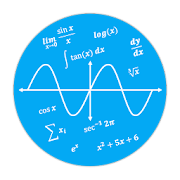# Calculator N+ (Open source) - Math SolverEveryone
11,954
The Calculator provides the following features:
ALGEBRA
1. Computer science
2. Solving the equation:
3. Solve system equations
4. Graphing
5. Cartesian geometry sand(Viet Nam Education)
6. Unit Conversions
8. Simplify expressions
9. Polynomial factorization.
10. Binomial expansion Newton
11. Matrix: evalute matrix step by step

ANALYTICS
1. Derivative
2. Antiderivative
3. Definite integrals
4. Find the limit of the sequence, function

TRIGONOMETRIC
1, Trigonometric expand: sin(2x) -> 2sin(x)cos(x)
2. Trigonometric reduce: 2sin(x)cos(x) -> sin(2x)
3. Trigonometric to exponent: sinh(x) -> (e^x-e^(-x))/2

DETERMINATION OF STATISTICS
1. Combination
2. Permutation

SOME OTHER FEATURES
1. Prime factors
2. Modulo
3. Catalan number
4. Fibonacci number

The Calculator does not show the steps to solve the equations, system of equations, derivative... but only for the final result.
Enter sin(30°) to calculate sine Degree mode

The computer can work in two modes:
- Decimal mode: 0.12312312323
- Mode fractions: for results with arbitrary precision, for example 9^99999

Fanpage application support: https://www.facebook.com/calculator.n.plus/
Any suggestions on the application please send mail: Tranleduy1233@gmail.com.

See in github https://github.com/tranleduy2000/ncalc
Read more
Collapse

Review Policy
4.7
11,954 total
5
4
3
2
1
Loading...

## What's New

Update math engine and documents
IDE mode
Read more
Collapse

## Additional Information

Updated
August 4, 2018
Size
6.9M
Installs
100,000+
Current Version
3.4.1
Requires Android
4.0.3 and up
Content Rating
Everyone
Permissions
Offered By
Mr Duy
Developer
Long Xuyen city, An Giang province, Viet Nam©2019 GoogleSite Terms of ServicePrivacyDevelopersArtistsAbout Google|Location: United StatesLanguage: English (United States)
By purchasing this item, you are transacting with Google Payments and agreeing to the Google Payments Terms of Service and Privacy Notice.